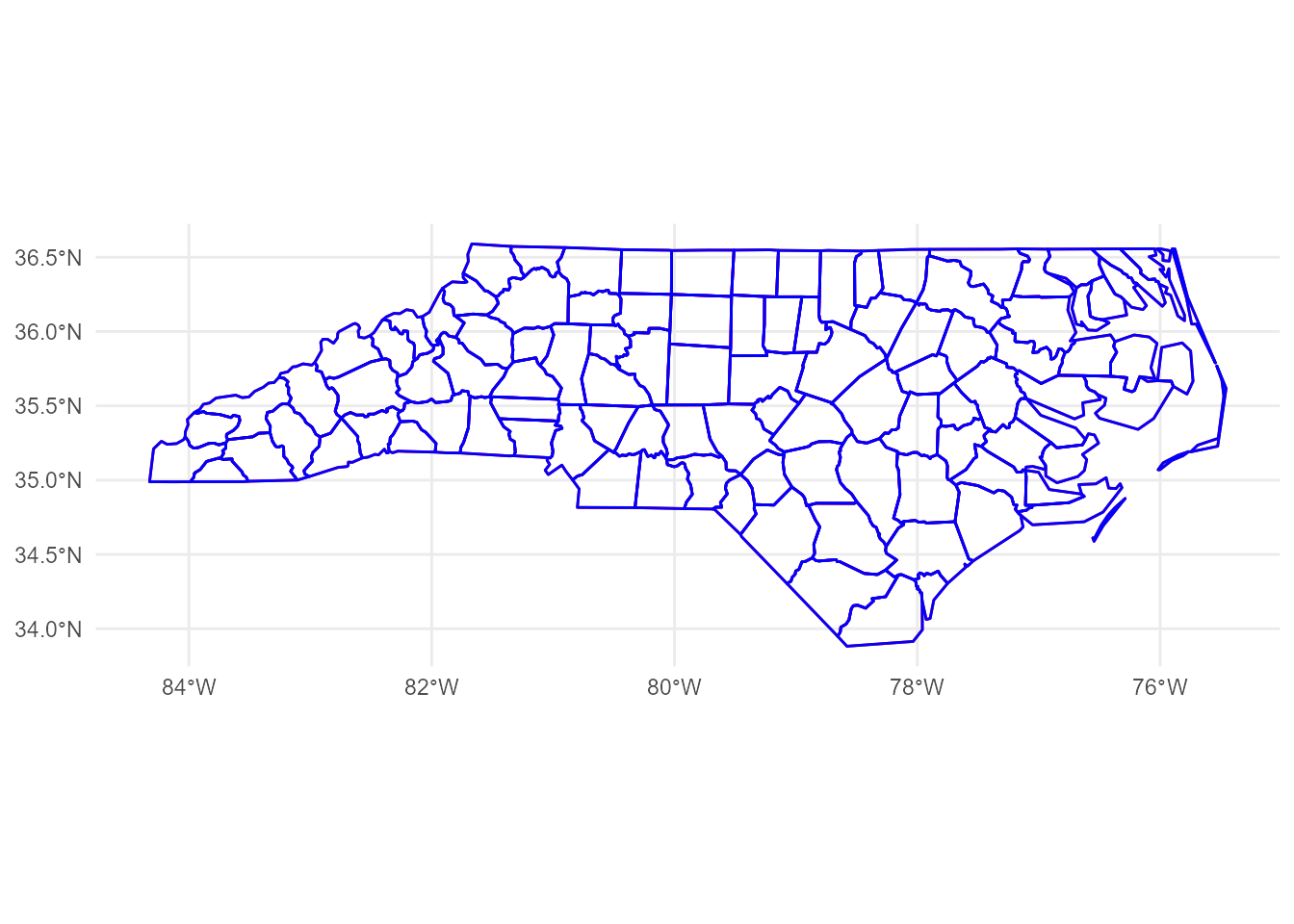# Plot geom_sf() On OpenStreetMap Tiles

ggplot2
GIS
Author

Hiroaki Yutani

Published

June 9, 2018

mapview is a very nice package to explore an `sf` object. It can overlay `sf` object on the map images:

``````nc <- sf::read_sf(system.file("shape/nc.shp", package="sf"))

# mapview::mapview(nc)``````

But, how can I do this with ggplot2? (My friend told me `mapview::mapshot()` can generate a PNG, but I want to do this with ggplot2!)

## RTFM

Before anything, I need to read Tile Usage Policy to use the OpenStreetMap tiles. For “Requirements” section, this is important:

``labs(caption = "\U00a9 OpenStreetMap contributors")``

For “Technical Usage Requirements” section, I have to read this more carefully. Let’s look at the requirements one by one.

Valid HTTP User-Agent identifying application. Faking another app’s User-Agent WILL get you blocked.

Oh, it seems I need to add `User-Agent` header. OK, let’s invent some nice name… If I use `httr::GET()`, the code will probably like this:

``````GET(
"https://...",
)``````

If known, a valid HTTP Referer.

I don’t have `Referer`s, so I skip this.

DO NOT send no-cache headers. (“Cache-Control: no-cache”, “Pragma: no-cache” etc.)

I do nothing other than swearing I’ll never use this header.

Ah, this is important. Let’s implement later.

Fortunately, I’m not good at parallelism, so this is fine.

## Get Tile URLs

According to Slippy map tilenames, the URL of a tile follows this format:

``https://[abc].tile.openstreetmap.org/zoom/x/y.png ``

I have to fill these four parts:

• `[abc]`
• `zoom`
• `x`
• `y`

Let’s look at these one by one.

### `[abc]`

`[abc]` means there are three domains; `a.tile.openstreetmap.org`, `b.tile.openstreetmap.org`, `c.tile.openstreetmap.org`. But, why? It says:

Browser-based applications can thus request multiple tiles from multiple subdomains faster than from one subdomain.

So, as I’m not browser-based, I can choose arbitrary one.

### `zoom`

Zoom parameter is an integer number from 0 to 19. If `zoom` is 0, there’s only one tile. Likewise 2 x 2 tiles for zoom `1`, 4 x 4 tiles for zoom `2`, and so on. Then, which one should I choose? This can be roughly determined based on the size of the bbox (boundary box) of the `sf` object.

``````# get the bbox
b <- sf::st_bbox(nc)
b``````
``````     xmin      ymin      xmax      ymax
-84.32385  33.88199 -75.45698  36.58965 ``````
``````# calculate the lengths of x and y of the bbox
x_len <- b["xmax"] - b["xmin"]
y_len <- b["ymax"] - b["ymin"]

# calculate the minimum zoom level that is smaller than the lengths
x_zoom <- sum(x_len < 360 / 2^(0:19)) - 1
y_zoom <- sum(y_len < 170.1022 / 2^(0:19)) - 1

zoom <- min(x_zoom, y_zoom)
zoom``````
`` 5``

But, since the tile is so small as 256 × 256 pixel, it’s often better to zoom more.

``zoom <- zoom + 2``

(I’m not sure how to do this correctly, I guess the zoom level should be determined by the size of the plot canvas, not the bbox.)

### `x` and `y`

A pair of `x` and `y` represents the location of a tile. `x` corresponds to longitude, `y` to latitude. If the `zoom` is given, I can convert longitudes and latitudes to `x` and `y` according to the pseudo code on OpenStreetMap’s wiki:

``````sec <- function(x) {
1 / cos(x)
}

lonlat2xy <- function(lat_deg, lon_deg, zoom) {
n <- 2^zoom

x <- (n * (lat_deg + 180)) %/% 360
lon_rad <- lon_deg * pi / 180
y <- (n * (1 - log(tan(lon_rad) + sec(lon_rad)) / pi)) %/% 2

list(x = x, y = y)
}``````

But, how can I find the set of tiles which covers the whole bbox?

``````library(ggplot2)

p <- ggplot(nc) +
geom_sf() +
annotate("rect", xmin = b["xmin"], xmax = b["xmax"], ymin = b["ymin"], ymax = b["ymax"],
colour = alpha("red", 0.4), fill = "transparent", linetype = "dashed", size = 1.2)``````
``````size aesthetic has been deprecated for use with lines as of ggplot2 3.4.0
This message is displayed once every 8 hours.``````
``p``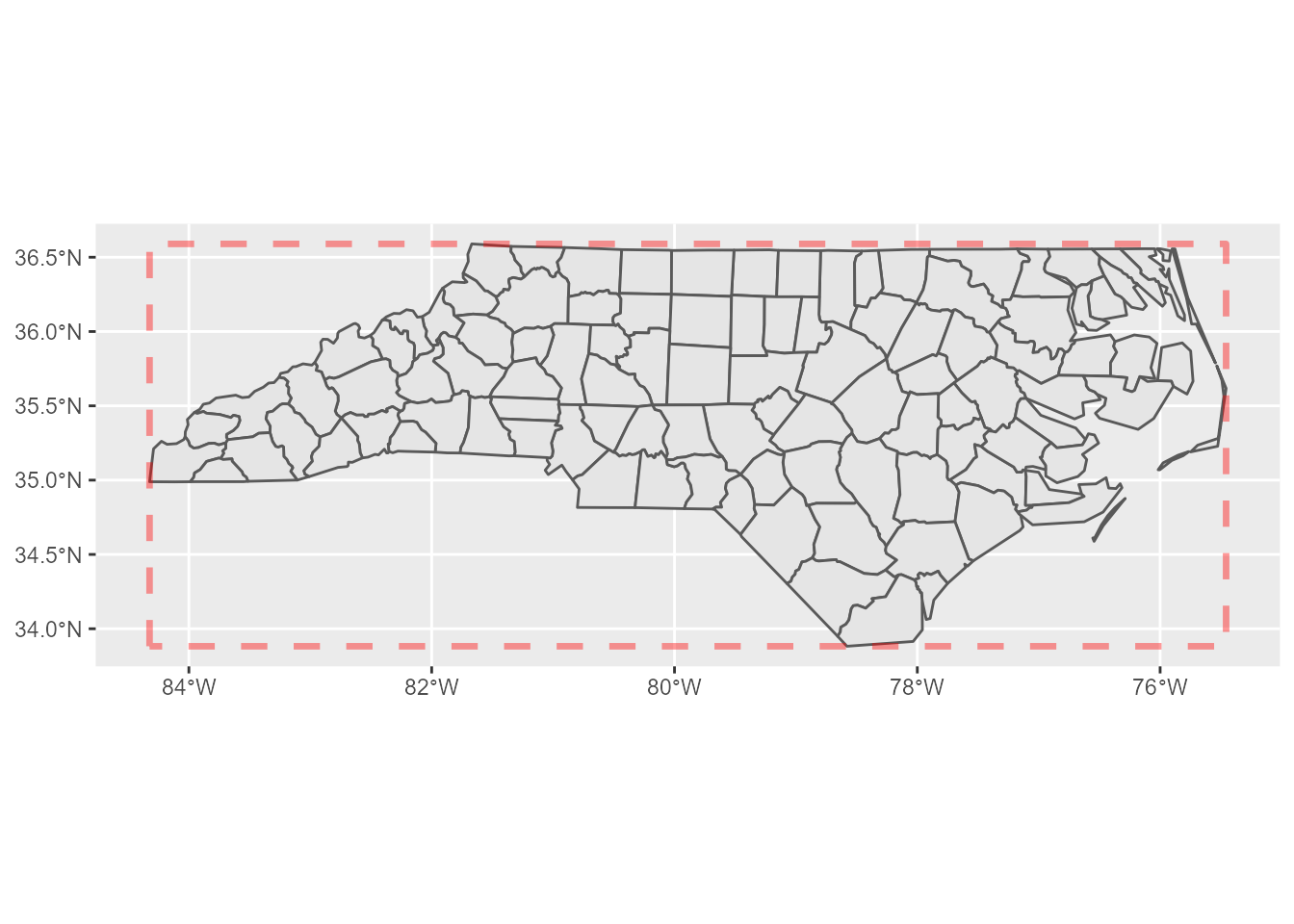This problem can be simplified; I can focus only two corners, the north-west and the south-east. If I calculate which tiles of `x` and `y` those two points fall in, I can find the rest of the tiles by filling the sequences of `x` and `y` between these two tiles.

``````corners <- expand.grid(x = b[c(1, 3)], y = b[c(2, 4)])

p +
geom_point(aes(x, y), corners[2:3,], colour = "red", size = 5)``````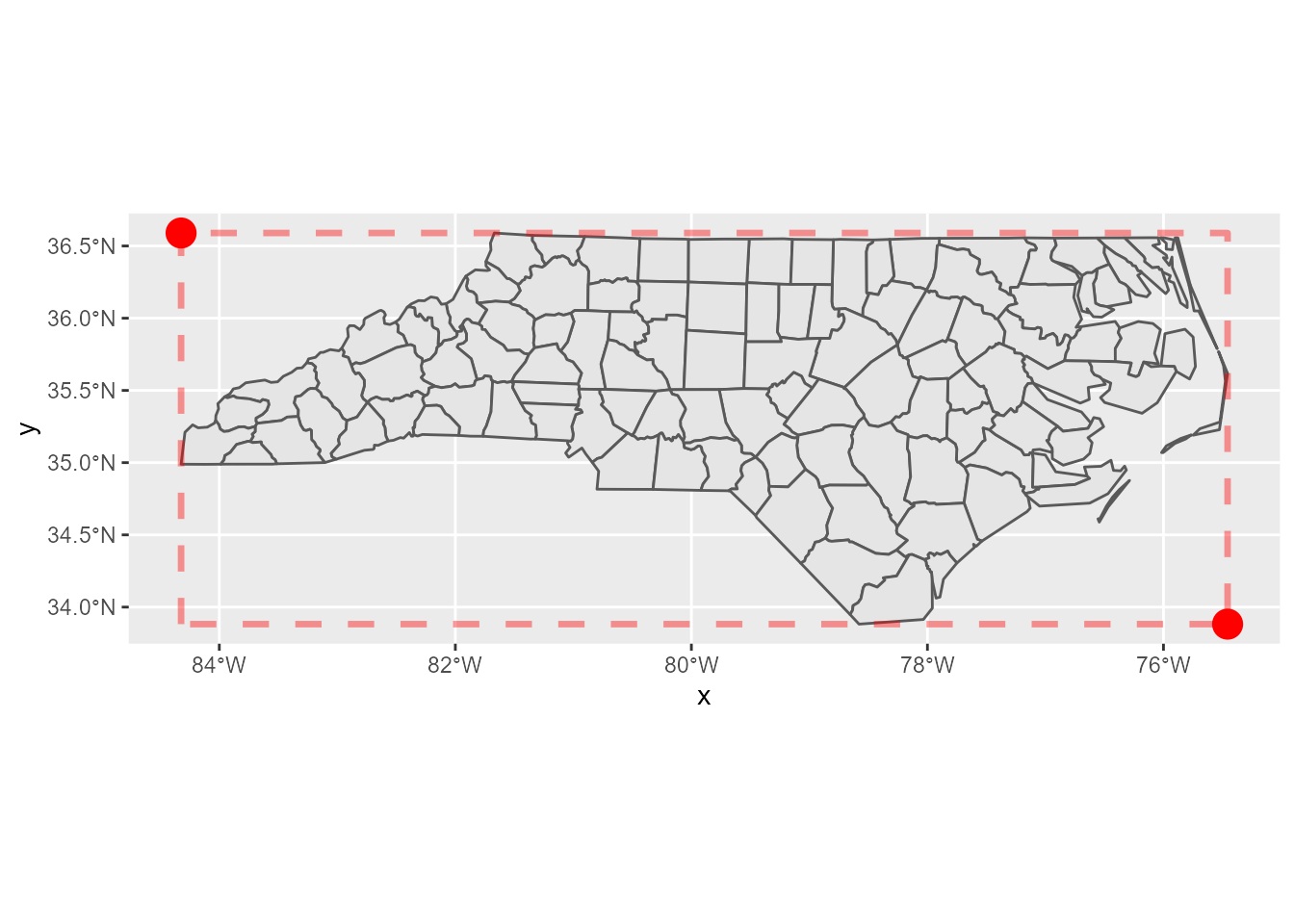Here’s the tiles:

``````xy <- lonlat2xy(b[c("xmin", "xmax")], b[c("ymin", "ymax")], zoom)

tiles <- expand.grid(x = seq(xy\$x["xmin"], xy\$x["xmax"]),
y = seq(xy\$y["ymin"], xy\$y["ymax"]))

tiles``````
``````   x  y
1 34 51
2 35 51
3 36 51
4 37 51
5 34 50
6 35 50
7 36 50
8 37 50``````

### Tile URLs

From the results above, I can yield the URLs of the tiles:

``````urls <- sprintf("https://a.tile.openstreetmap.org/%d/%d/%d.png", zoom, tiles\$x, tiles\$y)
urls``````
`````` "https://a.tile.openstreetmap.org/7/34/51.png"
 "https://a.tile.openstreetmap.org/7/35/51.png"
 "https://a.tile.openstreetmap.org/7/36/51.png"
 "https://a.tile.openstreetmap.org/7/37/51.png"
 "https://a.tile.openstreetmap.org/7/34/50.png"
 "https://a.tile.openstreetmap.org/7/35/50.png"
 "https://a.tile.openstreetmap.org/7/36/50.png"
 "https://a.tile.openstreetmap.org/7/37/50.png"``````

OK, now I can download them. But, before that, let’s confirm that the tiles really cover the expected area.

### Tile positions

To plot these tiles, I calculate the north-west corner of a tile by the following code (the pseudo code for this is also found on the wiki):

``````xy2lonlat <- function(x, y, zoom) {
n <- 2^zoom

lon_deg <- x / n * 360.0 - 180.0
lat_rad <- atan(sinh(pi * (1 - 2 * y / n)))
lat_deg <- lat_rad * 180.0 / pi

list(lon_deg = lon_deg, lat_deg = lat_deg)
}``````

Then, south-east corners can be also calculated easily. Let’s calculate the both corners and bind them to a data.frame.

``````library(purrr)
library(dplyr, warn.conflicts = FALSE)

nw_corners <- pmap_dfr(tiles, xy2lonlat, zoom = zoom)
# add 1 to x and y to get the south-east corners
se_corners <- pmap_dfr(mutate_all(tiles, `+`, 1), xy2lonlat, zoom = zoom)

names(nw_corners) <- c("xmin", "ymax")
names(se_corners) <- c("xmax", "ymin")

tile_positions <- bind_cols(nw_corners, se_corners)
tile_positions``````
``````# A tibble: 8 × 4
xmin  ymax  xmax  ymin
<dbl> <dbl> <dbl> <dbl>
1 -84.4  34.3 -81.6  32.0
2 -81.6  34.3 -78.8  32.0
3 -78.8  34.3 -75.9  32.0
4 -75.9  34.3 -73.1  32.0
5 -84.4  36.6 -81.6  34.3
6 -81.6  36.6 -78.8  34.3
7 -78.8  36.6 -75.9  34.3
8 -75.9  36.6 -73.1  34.3``````

Now I can plot the empty tiles as below:

``````p +
geom_point(aes(x, y), corners[2:3,], colour = "red", size = 5) +
geom_rect(data = tile_positions,
aes(xmin = xmin, xmax = xmax, ymin = ymin, ymax = ymax),
colour = "blue", fill = "transparent")``````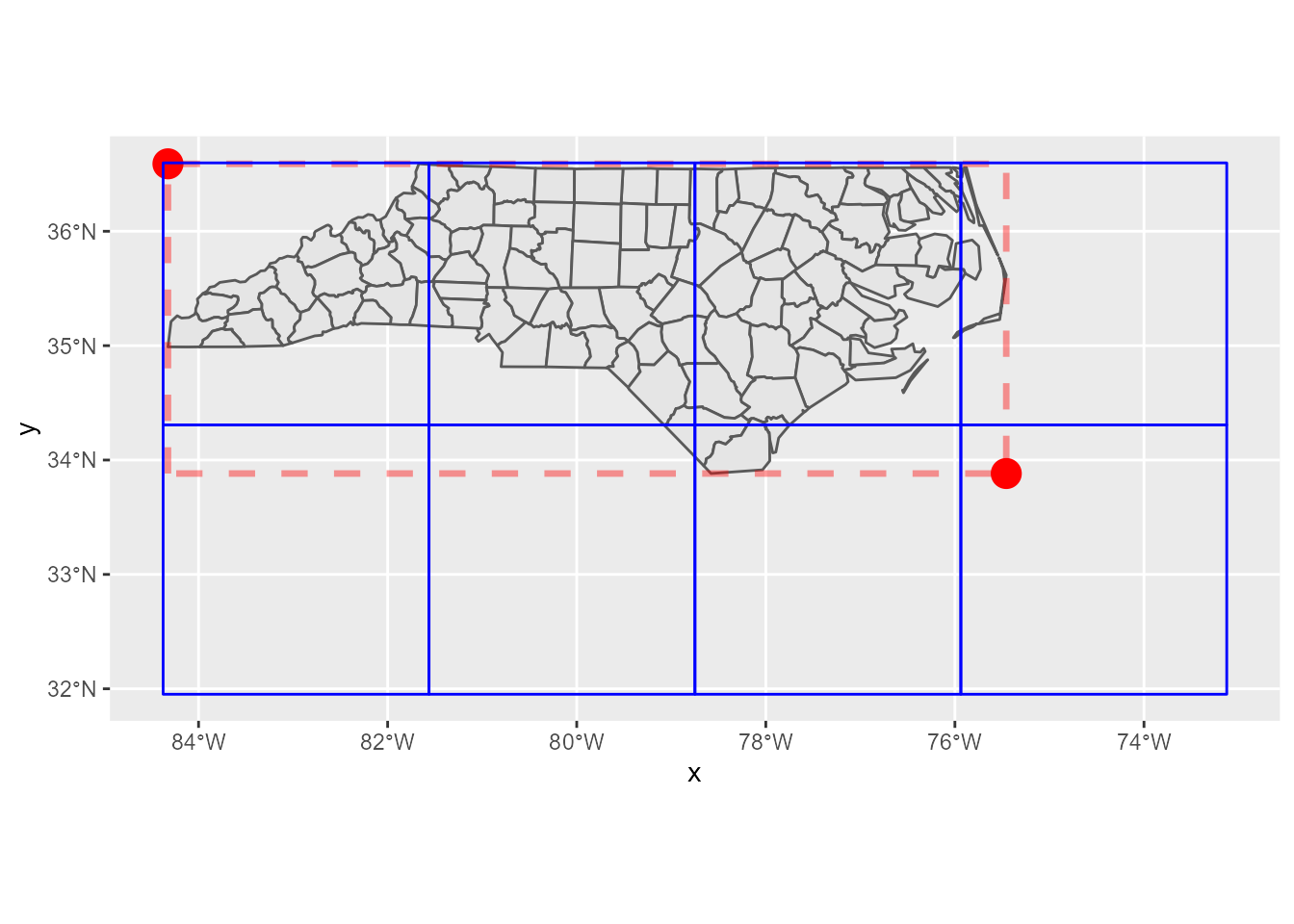Yay, confirmed! Let’s proceed to the next step.

## Get tile data

If I just get the response from a URL, `httr::GET()` is handy. But, this time, for the requirement of caching, I have to save the responses to disk first. So, I use `curl::curl_download()` here.

Note that PNG data can be read into R session by `png::readPNG()`.

``````get_tile <- function(url) {
# build a local path
path <- stringr::str_extract(url, "/\\d+/\\d+/\\d+.png")
local_png <- here::here(file.path("data", "osm-tiles", path))

if (!file.exists(local_png)) {
dir.create(dirname(local_png), showWarnings = FALSE, recursive = TRUE)

h <- curl::new_handle()
curl::handle_setheaders(h, `User-Agent` = "Yutani's blog post")

}

}``````

Then, let’s get all tiles.

``pngs <- map(urls, get_tile)``

## Plot tiles

To plot tiles, I use `annotation_raster()`, whose necessary arguments are:

• `raster`
• `xmin`
• `xmax`
• `ymin`
• `ymax`

The first one is `pngs` and the others are contained in `tile_positions`. So, let’s combine them so that I can use `pmap()`.

``````args <- tile_positions %>%
mutate(raster = pngs)

args``````
``````# A tibble: 8 × 5
xmin  ymax  xmax  ymin raster
<dbl> <dbl> <dbl> <dbl> <list>
1 -84.4  34.3 -81.6  32.0 <dbl [256 × 256 × 3]>
2 -81.6  34.3 -78.8  32.0 <dbl [256 × 256 × 3]>
3 -78.8  34.3 -75.9  32.0 <dbl [256 × 256 × 3]>
4 -75.9  34.3 -73.1  32.0 <dbl [256 × 256 × 3]>
5 -84.4  36.6 -81.6  34.3 <dbl [256 × 256 × 3]>
6 -81.6  36.6 -78.8  34.3 <dbl [256 × 256 × 3]>
7 -78.8  36.6 -75.9  34.3 <dbl [256 × 256 × 3]>
8 -75.9  36.6 -73.1  34.3 <dbl [256 × 256 × 3]>``````

Now I can plot tiles at last.

``````ggplot(nc) +
pmap(args, annotation_raster, interpolate = TRUE) +
geom_sf(fill = alpha("red", 0.3)) +
# don't forget the license notice!
labs(caption = "\U00a9 OpenStreetMap contributors") +
theme_minimal()``````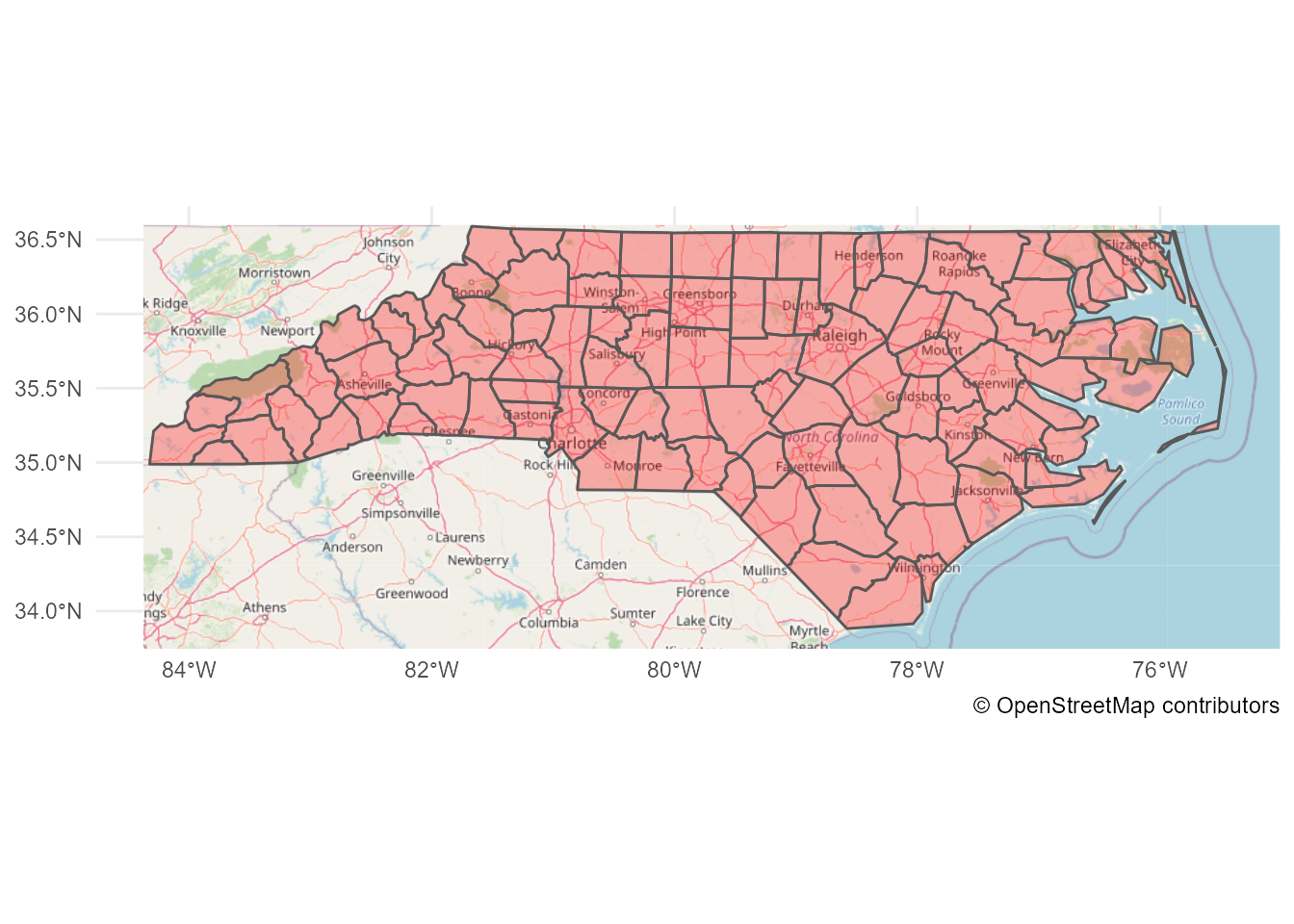Done!

But, I didn’t expect the code would be this long… Maybe I need to create a package for this.

## Caveats

Note that, I didn’t care about the CRS because `nc`’s CRS is fortunately EPSG 3857, which OpenStreetMap uses. If the `sf` object I want to plot has the different CRS, there may be a bit more to consider (and I don’t understand the CRS well…).

Update:

Sorry, I was wrong… `nc`’s CRS is EPSG 4267 and OpenStreetMap tiles use EPSG 4326. Thanks Edzer for pointing this out!

``sf::st_crs(nc)``
``````Coordinate Reference System:
wkt:
DATUM["North American Datum 1927",
ELLIPSOID["Clarke 1866",6378206.4,294.978698213898,
LENGTHUNIT["metre",1]]],
PRIMEM["Greenwich",0,
ANGLEUNIT["degree",0.0174532925199433]],
CS[ellipsoidal,2],
AXIS["latitude",north,
ORDER,
ANGLEUNIT["degree",0.0174532925199433]],
AXIS["longitude",east,
ORDER,
ANGLEUNIT["degree",0.0174532925199433]],
ID["EPSG",4267]]``````

So, I should have converted `nc` to the CRS first.

``nc_4326 <- sf::st_transform(nc, 4326)``

Fortunately, the difference between EPSG 4267 and EPSG 4326 is rather negligible for this scale, so the result map should look almost same if I used `nc_4326` instead of `nc`. Here’s the difference (can you see there’s a little red colors?):

``nc_4326_not_transformed <- sf::`st_crs<-`(nc, 4326)``
``````Warning: st_crs<- : replacing crs does not reproject data; use st_transform for
that``````
``````ggplot() +
geom_sf(data = nc_4326_not_transformed, fill = "transparent", colour = "red") +
geom_sf(data = nc_4326, fill = "transparent", colour = "blue") +
theme_minimal()``````